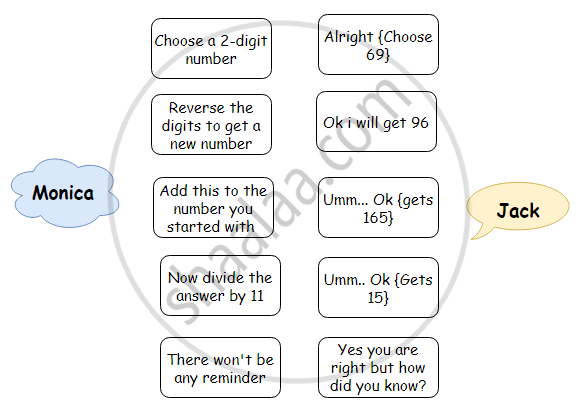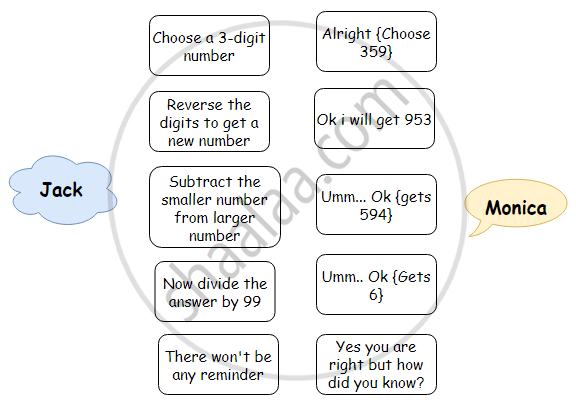# Games with Numbers

## Notes

### Games with Numbers:

There are several tricks with numbers to test divisibility by another numbers.

## (i) Reversing the digits - two digit number:Now, let us see if we can explain Monica’s “trick”. Suppose Jack chooses the number ab, which is a short form for the 2-digit number 10a + b. On reversing the digits, he gets the number ⇒ ba = 10b + a. When he adds the two numbers he gets ⇒ (10a + b) + (10b + a) = 11a + 11b = 11 (a + b). So, the sum is always a multiple of 11, just as Monica had claimed.

Similarly, we can try reversing the digits for dividing by 9.

Selecting a two-digit number: 85

Reversing the digits: 58

Subtract: 85 - 58 = 27

Divide by 9: 27/9 = 3

Remainder is 0.

 If the tens digit is larger than the ones digit (that is, a > b), he does: (10a + b) – (10b + a) = 10a + b – 10b – a = 9a – 9b = 9(a – b). If the ones digit is larger than the tens digit (that is, b > a), he does:(10b + a) – (10a + b) = 9(b – a). And, of course, if a = b, he gets 0.In each case, the resulting number is divisible by 9. So, the remainder is 0.

#### (ii) Reversing the digits – three digit number:Let us see how this trick works. Let the 3-digit number chosen by Monica be abc = 100a + 10b + c. After reversing the order of the digits, she gets the number cba = 100c + 10b + a. On subtraction: If a > c, then the difference between the numbers is (100a + 10b + c) – (100c + 10b + a) = 100a + 10b + c – 100c – 10b – a = 99a – 99c = 99(a – c). If c > a, then the difference between the numbers is (100c + 10b + a) – (100a + 10b + c) = 99c – 99a = 99(c – a). And, of course, if a = c, the difference is 0.In each case, the resulting number is divisible by 99. So the remainder is 0.

#### (iii) Forming three-digit numbers with given three-digits:

For a given three-digit number, rearrange the digits such that all the three numbers are distinct and add them all. Then dividing it by 37, the remainder is always 0.

For example, assuming a three-digit number 489.

On rearranging the digits: 894 and 948.

Add all the three numbers: 489 + 894 + 948 = 2331

Divide 2331 by 37: 2331/37 = 63; remainder is 0.

 Let us see. abc = 100a + 10b + c cab = 100c + 10a + b bca = 100b + 10c + a abc + cab + bca = 111(a + b + c) = 37 × 3(a + b + c), which is divisible by 37
If you would like to contribute notes or other learning material, please submit them using the button below.

### Shaalaa.com

Sum Difference Trick [00:06:53]
S
0%